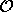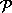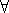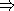## CGAL::Interval_nt

### Definition

This section describes briefly what interval arithmetic is, its implementation in CGAL, and its possible use by geometric programs. The main reason for having interval arithmetic in CGAL is its integration into the filtered robust and fast predicates scheme, but we also provide a number type so that you can use it separately if you find any use for it (such as interval analysis, or to represent data with tolerance).

The purpose of interval arithmetic is to provide an efficient way to bound the roundoff errors made by floating point computations. You can choose the behaviour of your program depending on these errors; that is what is done for the filtered robust predicates (see Section). You can find more theoretical information on this topic in [BBP01].

Interval arithmetic is a large concept and we will only consider here a simple arithmetic based on intervals whose bounds are doubles. So each variable is an interval representing any value inside the interval. All arithmetic operations (+, -, *, /, sqrt(), square(), min(), max() and abs()) on intervals preserve the inclusion. This property can be expressed by the following formula (x and y are reals, X and Y are intervals,is an arithmetic operation):xX,yY, (xy)(XY)

For example, if the final result of a sequence of arithmetic operations is an interval that does not contain zero, then you can safely determine its sign.

#include <CGAL/Interval_arithmetic.h>

FieldNumberType

### Creation

 Interval_nt I ( double d); introduces the interval [d;d]. Interval_nt I ( double i, double s); introduces the interval [i;s].

### Operations

All functions required by a class to be considered as a CGAL number type (see) are present, as well as the utility functions, sometimes with a particular semantic which is described below. There are also a few additional functions.

 Interval_nt I / J returns [-;+] when the denominator contains 0. Interval_nt sqrt ( I) returns [0;sqrt(upper_bound(I))] when only the lower bound is negative (expectable case with roundoff errors), and is unspecified when the upper bound also is negative (unexpected case). double to_double ( I) returns the middle of the interval, as a double approximation of the interval. double I.inf () returns the lower bound of the interval. double I.sup () returns the upper bound of the interval. bool I.is_point () returns whether both bounds are equal. bool I.is_same ( J) returns whether both intervals have the same bounds. bool I.do_overlap ( J) returns whether both intervals have a non empty intersection.

The two following operators can be used for interval analysis, they are not directly useful for Interval_nt as a number type.

 Interval_nt I || J returns the smallest interval containing the two intervals. Interval_nt I && J returns the biggest interval contained in the two intervals. The result is unspecified if the two intervals don't intersect.

The comparison operators (<, >, <=, >=, ==, !=, sign() and compare()) have the following semantic: it is the intuitive one when for all couples of values in both intervals, the comparison is identical (case of non-overlapping intervals). This can be expressed by the following formula (x and y are reals, X and Y are intervals,is a comparison operator):

 (xX,yY, (xy) = true)(XY) = true

and

 (xX,yY, (xy) = false)(XY) =false

Otherwise, the comparison is not safe, and we first increment the counter Interval_nt_advanced::number_of_failures, and then throw the exception Interval_nt_advanced::unsafe_comparison.

### Implementation

Interval_nt derives from Interval_nt_advanced. The operations on Interval_nt are automatically protected against rounding modes, and are thus slower than those on Interval_nt_advanced, but easier to use.

Users that need performance are encouraged to use Interval_nt_advanced instead (see Section).